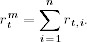3.15 Alternative Approaches

In this section, we discuss two alternative methods to volatility modeling.

3.15.1 Use of High-Frequency Data

French, Schwert, and Stambaugh (1987) consider an alternative approach for volatility estimation that uses high-frequency data to calculate volatility of low-frequency returns. In recent years, this approach has attracted substantial interest due to the availability of high-frequency financial data; see Andersen, Bollerslev, Diebold, and Labys (2001a, 2001b).

Suppose that we are interested in the monthly volatility of an asset for which daily returns are available. Letbe the monthly log return of the asset at month t. Assume that there are n trading days in month t and the daily log returns of the asset in the month are. Using properties of log returns, we haveAssuming that the conditional variance and covariance exist, we have

(3.48)where Ft−1 denotes the information available at month t − 1 (inclusive). The prior equation can be simplified if additional assumptions are made. For example, if we assume that {rt, i} is a white noise series, then ...

Get Analysis of Financial Time Series, Third Edition now with the O’Reilly learning platform.

O’Reilly members experience books, live events, courses curated by job role, and more from O’Reilly and nearly 200 top publishers.